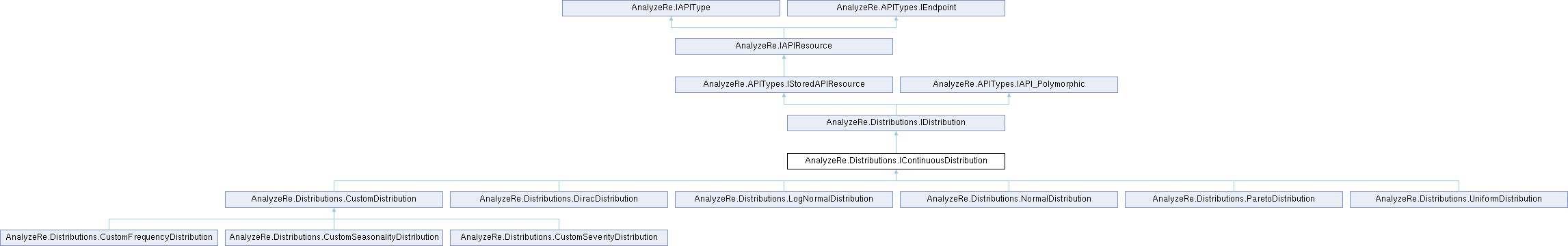C# Client Library A C# Client Library for the AnalyzeRe REST API
AnalyzeRe.Distributions.IContinuousDistribution Interface Reference

Represents any distribution that is continuous. With a continuous probability distribution, the probability that a random variable will assume a particular value is zero. As such, these distributions cannot be expressed in tabular form and must be described by an equation. More...

## Public Member Functions

ShallowCopy< T > ()
Create a shallow copy of this object. More...

## Properties

string collection_name` [get]`
The relative path from the root of the API at which the collection of resources of this type reside. More...

DateTime created` [get, set]`
The date and time when the resource was created on the system. More...

string description` [get, set]`
A user-friendly description of the resource. More...

string id` [get, set]`
The resource's unique identifier. It will be used in the request URL when requesting the resource from the server. More...

double max_val` [get, set]`
The server calculated maximum value of this distribution (if available) More...

double mean_val` [get, set]`
The server calculated mean value of this distribution (if available) More...

Dictionary< string, object > meta_data` [get, set]`
Any user-defined metadata for this layer. More...

double min_val` [get, set]`
The server calculated minimum value of this distribution (if available) More...

DateTime modified` [get, set]`
The date and time when the resource was last modified on the system. More...

double modulo` [get, set]`
Sets a maximum value for the distribution. Any values greater than or equal to this will be wrapped back to zero. (For example, if modulo = 365, when the distribution produces a value of 370.5, the resulting value will be 5.5, and a value of exactly 365 would wrap to 0.) Similarly, negative numbers produced by the distribution will get wrapped up to a number between 0 and modulo. Leave modulo equal to null and distribution values will remain unmodified. More...

string Path` [get]`
The relative path to this endpoint from the root URL. More...

string type` [get]`
The server identifier for this resource's polymorphic sub-type. More...

## Detailed Description

Represents any distribution that is continuous. With a continuous probability distribution, the probability that a random variable will assume a particular value is zero. As such, these distributions cannot be expressed in tabular form and must be described by an equation.

Definition at line 7 of file IContinuousDistribution.cs.

Inheritance diagram for AnalyzeRe.Distributions.IContinuousDistribution:## Member Function Documentation

 T AnalyzeRe.IAPIType.ShallowCopy< T > ( )
inherited

Create a shallow copy of this object.

Returns
A shallow copy of this object.

Implemented in AnalyzeRe.APIType.

Type Constraints
 T : IAPIType

## Property Documentation

 string AnalyzeRe.IAPIResource.collection_name
getinherited

The relative path from the root of the API at which the collection of resources of this type reside.

Definition at line 19 of file IAPIResource.cs.

 DateTime AnalyzeRe.APITypes.IStoredAPIResource.created
getsetinherited

The date and time when the resource was created on the system.

Definition at line 19 of file IStoredAPIResource.cs.

 string AnalyzeRe.APITypes.IStoredAPIResource.description
getsetinherited

A user-friendly description of the resource.

Definition at line 14 of file IStoredAPIResource.cs.

 string AnalyzeRe.IAPIResource.id
getsetinherited

The resource's unique identifier. It will be used in the request URL when requesting the resource from the server.

Definition at line 14 of file IAPIResource.cs.

 double AnalyzeRe.Distributions.IDistribution.max_val
getsetinherited

The server calculated maximum value of this distribution (if available)

Definition at line 28 of file IDistribution.cs.

 double AnalyzeRe.Distributions.IDistribution.mean_val
getsetinherited

The server calculated mean value of this distribution (if available)

Definition at line 24 of file IDistribution.cs.

 Dictionary AnalyzeRe.APITypes.IStoredAPIResource.meta_data
getsetinherited

Any user-defined metadata for this layer.

Definition at line 30 of file IStoredAPIResource.cs.

 double AnalyzeRe.Distributions.IDistribution.min_val
getsetinherited

The server calculated minimum value of this distribution (if available)

Definition at line 20 of file IDistribution.cs.

 DateTime AnalyzeRe.APITypes.IStoredAPIResource.modified
getsetinherited

The date and time when the resource was last modified on the system.

Definition at line 24 of file IStoredAPIResource.cs.

 double AnalyzeRe.Distributions.IDistribution.modulo
getsetinherited

Sets a maximum value for the distribution. Any values greater than or equal to this will be wrapped back to zero. (For example, if modulo = 365, when the distribution produces a value of 370.5, the resulting value will be 5.5, and a value of exactly 365 would wrap to 0.) Similarly, negative numbers produced by the distribution will get wrapped up to a number between 0 and modulo. Leave modulo equal to null and distribution values will remain unmodified.

Definition at line 16 of file IDistribution.cs.

 string AnalyzeRe.APITypes.IEndpoint.Path
getinherited

The relative path to this endpoint from the root URL.

Definition at line 13 of file IEndpoint.cs.

 string AnalyzeRe.APITypes.IAPI_Polymorphic.type
getinherited

The server identifier for this resource's polymorphic sub-type.

Definition at line 10 of file IAPI_Polymorphic.cs.

The documentation for this interface was generated from the following file: